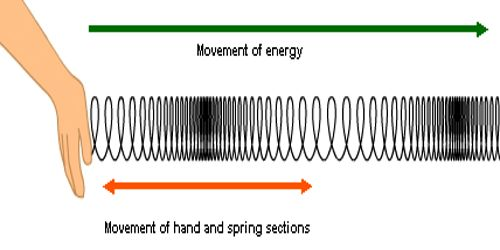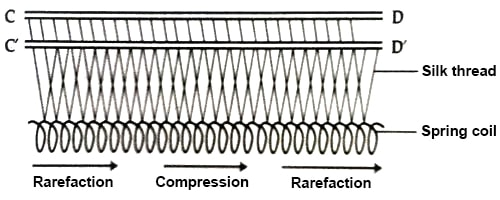# Experiment: Demonstration of Longitudinal Wave

Experiment: Demonstration of longitudinal wave

In this experiment a helical coil of thin wire containing several turns is hung from two horizontal rods CD and CꞌDꞌ by a fine silk thread in V-shape. The hanging of the coil is such that it remains horizontal [Figure]Holding one end of the coil of spring by hand if a sudden horizontal push is given to the coil it will be observed that the coil is compressed and elongated successively and this vibration gradually progresses along the line at the spring. That means, the coil of the spring vibrates along the propagation of wave executing simple harmonic motion. So the generated wave is the longitudinal wave.

In a longitudinal wave the particle displacement is parallel to the direction of wave propagation. The wavelength in a longitudinal wave refers to the distance between two consecutive compressions or between two consecutive rarefactions. The amplitude is the maximum displacement from a position of rest. The amplitude is the distance from the equilibrium position of the medium to a compression or a rarefaction.